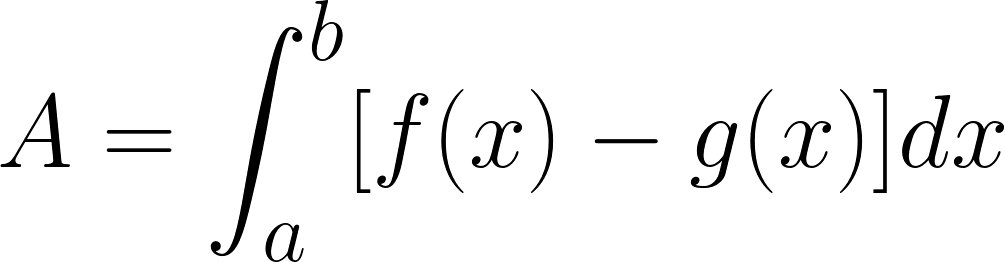### 5.A. Area Between Two Graphs

With a few modifications, you can extend the application of definite integrals from the area of a region under a curve to the area of a region between two curves.• Key Terms
• definite integral
• antidifferentiation
• antiderivative

Essential Questions
• How can definite integrals be used to find the area of a region bounded by two curves?
Skills
• Find the area of a region between two curves using integration.
• Find the area of a region between intersecting curves using integration.
• Describe integration as an accumulation process.